Courses

# Circles - Olympiad Level MCQ, Class 9 Mathematics

## 25 Questions MCQ Test Olympiad Preparation for Class 9 | Circles - Olympiad Level MCQ, Class 9 Mathematics

Description
This mock test of Circles - Olympiad Level MCQ, Class 9 Mathematics for Class 9 helps you for every Class 9 entrance exam. This contains 25 Multiple Choice Questions for Class 9 Circles - Olympiad Level MCQ, Class 9 Mathematics (mcq) to study with solutions a complete question bank. The solved questions answers in this Circles - Olympiad Level MCQ, Class 9 Mathematics quiz give you a good mix of easy questions and tough questions. Class 9 students definitely take this Circles - Olympiad Level MCQ, Class 9 Mathematics exercise for a better result in the exam. You can find other Circles - Olympiad Level MCQ, Class 9 Mathematics extra questions, long questions & short questions for Class 9 on EduRev as well by searching above.
QUESTION: 1

Solution:
QUESTION: 2

Solution:
QUESTION: 3

### A chord of a circle is 12 cm in length and its distance from the centre is 8 cm. Find the length of the chord of the same circle which is at a distance of 6 cm from the centre.

Solution:
QUESTION: 4

Which of the following statement(s) is / are true ?

Solution:
QUESTION: 5

In the given figure, find the value of x.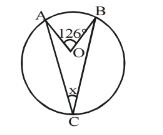Solution:
QUESTION: 6

In the given figure, ΔABC is inscribed in a circle with centre O. If ∠ACB = 65°, find ∠ABC.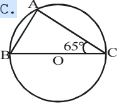Solution:
QUESTION: 7

In the given figure if O is the center of the circle, then find x.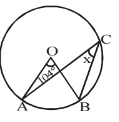Solution:
QUESTION: 8

An equilateral triangle PQR is inscribed in a circle with centre O. Find ∠QOR.

Solution:
QUESTION: 9

In the given figure, ΔXYZ is inscribed in a circle with centre O. If the length of chord YZ is equal to the radius of the circle OY then ∠YXZ =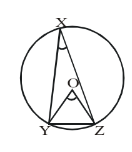Solution:
QUESTION: 10

In the given figure, O is the centre of a circle. If ∠DAC = 54° and ∠ACB = 63° then ∠BAC =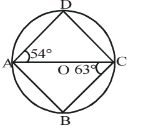Solution:
QUESTION: 11

In the given figure, AOB is a diameter of a circle with centre O. If ∠BOD = 120°, find ∠ACD.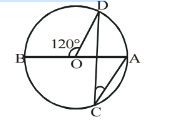Solution:
QUESTION: 12

AB is a chord of a circle with centre O and radius 17 cm. If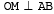and OM = 8 cm, find the length of chord AB.

Solution: 30
ab=2am
=2( 17^2-8^2)sq root
=2×15=30
QUESTION: 13

In the given figure, O is the centre of the circle. Find the value of x.Solution:

Angle BDC = angle BAC. ( Angles on same segment)
In ∆BDC, by angle sum property of a triangle,
angle( DBC + BDC + DCB) = 180′
60' + 45' + 40' + angle OCD = 180'
145' + angle OCD = 180'
angle OCD = 180' - 145'
angle OCD = 35'

Angle BCD = 35' + 40'
x = 75'

QUESTION: 14

In the given figure, ABCD is a quadrilateral incribed in a circle. Diagonals AC and BD are joined. If ∠CAD = 50° and ∠BDC = 45°. Find ∠BCD.Solution:
QUESTION: 15

Find the value of x ( ∠BCD) in the given figure.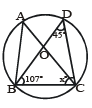Solution:
QUESTION: 16

In the given figure, two chords AB and CD of a circle intersect each other at a point E such that ∠BAC = 45°, ∠BED = 120°. Then find ∠ABD.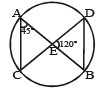Solution:
QUESTION: 17

In the given figure, AOB and COD are two diameters of a circle with centre O. If ∠OAC = 60°. Find ∠ABD.Solution:
QUESTION: 18

In the given figure, ABCD is a quadrilateral inscribed in a circle. Diagonals AC and BD are joined. If ∠CAD = 40° and ÐBDC = 25°. Find ∠BCD.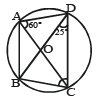Solution:
QUESTION: 19

Two chords AB and CD of a circle cut each other when produced outside the circle at P. AD and BC are joined. If ∠PAD = 30° and ∠CPA = 45°. Find ∠CBP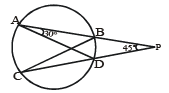Solution: BD is common arc it makes an angel DAB=angel DCB=30 then In triangle CBP 30+45=75 then 180–75=105 then angel CBP=105
QUESTION: 20

In the given figure, DABC is inscribed in a circle. The bisector of ∠BAC meets BC at D and the circle at E. If EC is joined then ∠ECD = 30°. Find ∠BAC.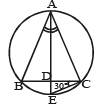Solution:
QUESTION: 21

In the given figure, ABCD is a cycle quadrilateral in which ∠BAD = 120°. Find ∠BCD.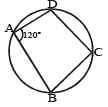Solution:
QUESTION: 22

In the given figure POQ is a diameter of a circle with centre O and PQRS is a cyclic quadrilateral. SQ is joined. If ∠R = 138°, find ∠PQS.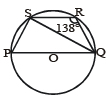Solution: We know that sum of opp angle of quad is 180
angle QRS+angle QPS=180
QPS=42
angle PSQ=90(angle in the semicircle is a right angle)
in triangle PQS 90+42+PQS=180
PQS=180_138=48
QUESTION: 23

In the given figure, PQRS is a cyclic trapezium in which PQ || SR. If ∠P = 82°. Find ∠S.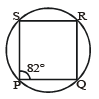Solution:
QUESTION: 24

Two circles intersect in A and B. Quadrilaterals PCBA and ABDE are inscribed in these circles such that PAE and CBD are line segment. If ∠P = 95° and ∠C = 40°. Find the value of Z.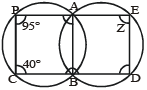Solution:
QUESTION: 25

Which of the following statement(s) is / are true ?

Solution: